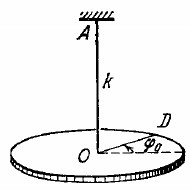# An arrangement illustrated in Fig consists of a horizontal uniform disc D of mass m and radius R and a thin rod AO whose torsional coefficient is equa...

An arrangement illustrated in Fig consists of a horizontal uniform disc D of mass m and radius R and a thin rod AO whose torsional coefficient is equal to k. Find the amplitude and the energy of small torsional oscillations if at the initial moment the disc was deviated through an angle φ, from the equilibrium position and then imparted an angular velocity φo.Anonymous User Physics Oscillation 02 May, 2020 55 views